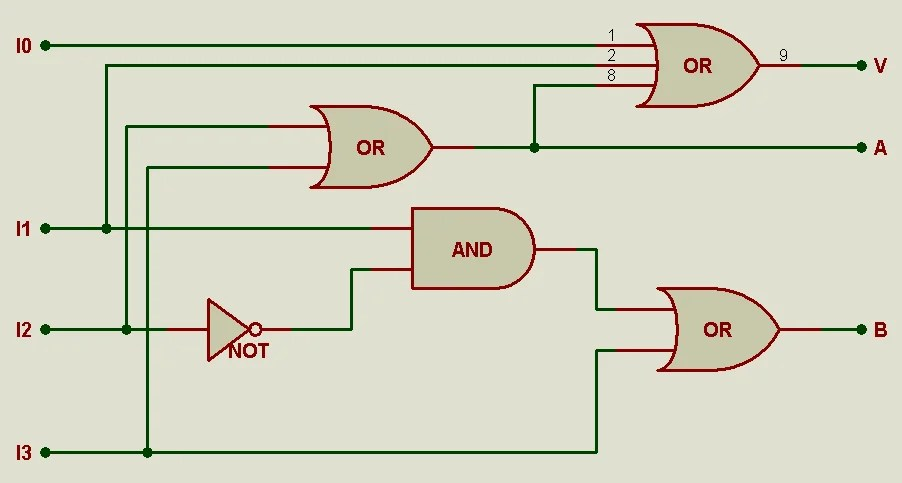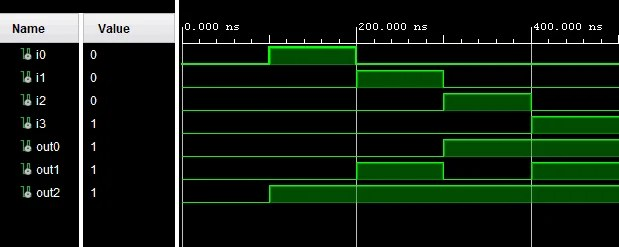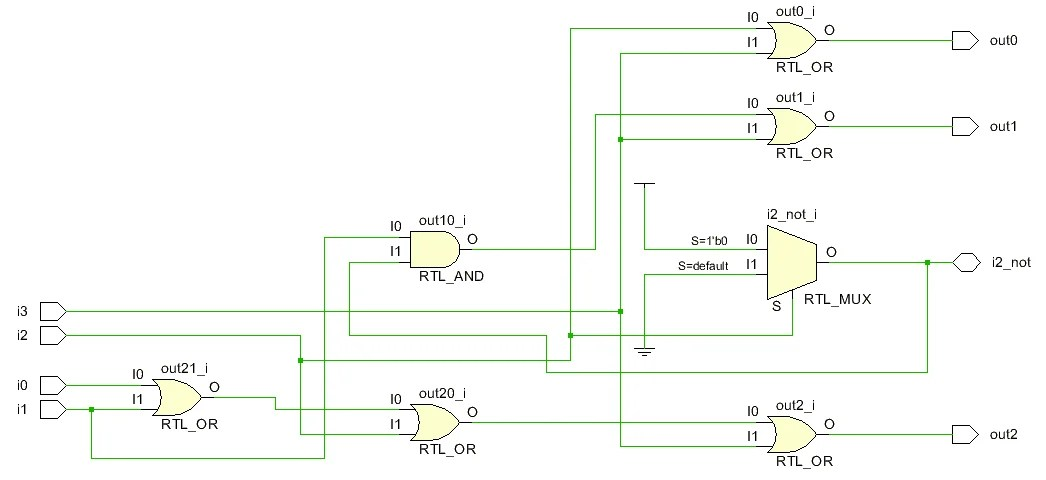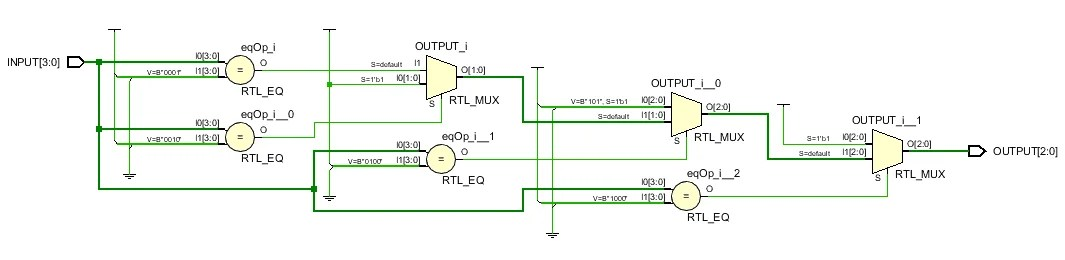View Course Path

# VHDL code for a priority encoder – All modeling styles

We will implement the VHDL code for a 4:2 Priority Encoder using three architectures, described by the three styles of modeling: Dataflow Modeling, Behavioral Modeling, Structural Modeling. We will also look at three different styles of writing the testbench for the circuit.

Contents

## Priority Encoder 4:2

A Priority Encoder is a circuit that compiles multiple binary inputs to a smaller number of outputs. It assigns a priority to the input and gives an output based on that priority. It is a modified version of an encoder. Let’s try to understand the functioning of a priority encoder with an example.

Consider a 4:2 Priority encoder

Number of inputs = 2^n & Number of outputs = n (n=1,2,3,4….)

The Priority encoders here are shown to have one more output pin. The extra pin is to indicate if the encoder is currently showing an output.Outputs are depicted as three in total – A, B, V. The ‘A’ and ‘B’ outputs are the ones truly required, but the V output serves an important purpose of checking if there is input signal at any input pins. Such a function is not important in small scale implementations of a Priority Encoder but is essential in large scale implementations.

### Truth table

The truth table contains variables I3, I2, I1, I0, and A, B, V. The letter ‘X’ denotes a “Don’t care” condition, in which the value of the bit is made irrelevant.

At a glance, we can understand the meaning of the truth table. Simply speaking, as we move from LSB to MSB, the priority of the input increases, and the value of the previous input bit becomes irrelevant.

 I3 I2 I1 I0 A B V 0 0 0 0 X X 0 0 0 0 1 0 0 1 0 0 1 X 0 1 1 0 1 X X 1 0 1 1 X X X 1 1 1

### Equations

Using the truth table equations can be derived for A, B, V.

•$A\quad =\quad I2\quad\cdot\quad \overline {I3} +\quad I3$
•$A \quad = \quad ( \quad I2 \quad + \quad I3 \quad ) \quad \cdot \quad ( \quad \overline {I3} \quad +\quad I3 \quad )$
•$A\quad =\quad I2\quad +\quad I3$

•$B\quad =\quad I1\quad\cdot\quad \overline {I2} \quad\cdot\quad \overline {I3} \quad +\quad I3$
•$B\quad =\quad (\quad I1\quad\cdot\quad I2\quad +\quad I3\quad )\quad\cdot\quad (\quad \overline {I3} \quad +\quad I3\quad )$
•$B\quad =\quad I1\quad\cdot\quad \overline {I2} \quad +\quad I3$

•$V \quad=\quad I0 \quad + \quad I1 \quad + \quad I2 \quad + \quad I3 \quad$

### Circuit diagram## Dataflow Modeling

The dataflow model executes in parallel. In dataflow modeling operators such as AND, OR, +, -, etc. can be used.

### VHDL code for a Priority Encoder using Dataflow Modeling

Declaring the entity and architecture, the entity for 4:2 encoder is Priority_encoder_A. Here we have declared five input variables i0, i1, i2, i3 and one inout STD_LOGIC variablei2_not to store the complement of i2. Three output variables out0, out1, out2. All the inputs and outputs are 1-bit variables.

entity Priority_encoder_A is
-- Declaring input and output variables
Port (
i0 : in STD_LOGIC;
i1 : in STD_LOGIC;
i2 : in STD_LOGIC;
i3 : in STD_LOGIC;
i2_not : inout STD_LOGIC;
out0 : out STD_LOGIC;
out1 : out STD_LOGIC;
out2 : out STD_LOGIC);
end Priority_encoder_A;

architecture Dataflow of Priority_encoder_A is
begin
process (i2) --get the complement of i2
begin
if i2 = '0' then
i2_not <= '1';
else
i2_not <= '0';
end if;
end process;

out2 <= i0 OR i1 OR i2 OR i3;    --Using Equation V
out1 <= (i1 AND i2_not) OR i3 ;  --Using Equation B
out0 <= i2 OR i3;                --Using Equation A
end Dataflow;

With the use of a simple process(i2) we get the complement of i2 and store it in i2_not.

### Output waveform for dataflow modeling## Behavioral Modeling

Programming the architecture using Behavioral modeling allows the code to run sequentially. As the name ‘behavioral’ suggests, we will be focussing on how the circuit ‘behaves’ on the application of inputs. In short, the circuit’s outputs on the application of a certain set of predetermined inputs.

### VHDL code for a Priority Encoder using Behavioral Modeling.

Unlike the previous program with fixed equations derived from the truth tables, we will use the truth table itself. A truth table, as you know, is the most elementary definition of the behavior of a circuit.

The entity for 4:2 Priority Encoder consists of two logic vector variables. INPUT is a ‘4-bit’ logic vector variable and OUTPUT is ‘3-bit’ logic vector variable. Considering the truth table INPUT contains variables i3,  i2,  i1,  i0 in that order. OUTPUT contains Variables A, B, V in that order.

 I3 I2 I1 I0 A B V 0 0 0 0 X X 0 0 0 0 1 0 0 1 0 0 1 X 0 1 1 0 1 X X 1 0 1 1 X X X 1 1 1

The entity for (4:2) Priority Encoder is,

entity Priority_Encoder_A is
Port ( INPUT : in STD_LOGIC_VECTOR (3 downto 0);
OUTPUT : out STD_LOGIC_VECTOR (2 downto 0));
end Priority_Encoder_A;

For the test bench of the 4:2 Priority Encoder, we are using all the possible cases for verification. Thus in the architecture, we will just check each position in the vector for the input of 1-bit. Depending on the position of the 1-bit input, the priority is assigned.

architecture Behavioral of Priority_Encoder_A is

begin
process(INPUT)
begin
if (INPUT(3)='1') then
OUTPUT <= "111";
elsif (INPUT(2)='1') then
OUTPUT <= "101";
elsif (INPUT(1)='1') then
OUTPUT <= "011";
elsif (INPUT(0)='1') then
OUTPUT <= "001";
else
OUTPUT <= "000";
end if;
end process;

end Behavioral;

### Output waveform for behavioral modeling## Structural Modeling

Structural modeling is using smaller modules to form a large module. The number of times smaller modules are used within the larger modules are known as instances. There can be multiple instances of the same smaller module within the larger module.

To make a 4:2 Priority Encoder, we can use multiple logic gate modules. Referring to the 4:2 Priority Encoder logic gate design, we can create the logic gates as modules (dataflow model).

The lines connecting the modules are called ‘Signals.’ These signals are dedicated for data transfer from one internal module to another or from module to an external point on the main module.

According to the logic gate design we require,

• AND Gate
• OR Gate
• NOT Gate
• OR Gate (3 inputs to 1 output)

First, we need to create the entity for 4:2 Priority Encoder, declare all the inputs and outputs required.

### VHDL code for Priority Encoder using Structural Modeling.

Let’s have a look at the VHDL code; we will use the dataflow model to define the smaller modules.

entity Priority_encoder is
Port (
i0 : in STD_LOGIC;
i1 : in STD_LOGIC;
i2 : in STD_LOGIC;
i3 : in STD_LOGIC;
out0 : out STD_LOGIC;
out1 : out STD_LOGIC;
out2 : out STD_LOGIC);
end Priority_encoder;

Within the architecture, we also need to define the components and the number of components/modules we require.

But first, we need the modules, so let’s create the entity and architecture of the Logic Gates.

These logic gate entities and architectures do not require test benches as they are part of a larger module and the larger module is subjected to testing from the testbench, so will we create a testbench for just the larger module.

VHDL code for Logic Gates

AND Gate

entity and_gate is
Port (
a_and : in STD_LOGIC;
b_and : in STD_LOGIC;
c_and : out STD_LOGIC);
end and_gate;

architecture Dataflow of and_gate is
begin
c_and <= a_and AND b_and;
end Dataflow;

OR Gate

entity or_gate is
Port (
a_or : in STD_LOGIC;
b_or : in STD_LOGIC;
c_or : out STD_LOGIC);
end or_gate;

architecture Dataflow of or_gate is
begin
c_or <= a_or OR b_or;
end Dataflow;

NOT Gate

entity not_gate is
Port (
a_not : in STD_LOGIC;
b_not : out STD_LOGIC);
end not_gate;

architecture Dataflow of not_gate is
begin
b_not <= NOT a_not;
end DataFlow;

OR Gate (3 inputs to 1 output)

entity or3_gate is
Port (
a_or3 : in STD_LOGIC;
b_or3 : in STD_LOGIC;
c_or3 : in STD_LOGIC;
d_or3 : out STD_LOGIC);
end or3_gate;

architecture Dataflow of or3_gate is
begin
d_or3 <= a_or3 OR(b_or3 OR c_or3);
end Dataflow;

#### VHDL code for 4:2 Priority Encoder

The entity will simply consist of the required inputs and outputs. We will use inputs i0, i1,i2, i3, and outputs out0, out1, out2. All the inputs and outputs are declared as Standard Logic here.

The architecture is where we declare all the instances of the component required. Here we require two OR gates labeled or0 & or1, one AND Gate labeled and0, one NOT Gate labeled not0 and one OR Gate (3 inputs to 1 output) labeled or_3.

Remember to use formal port mapping. As the complexity of the program increases, it is much easier to keep track of the modules with the use of formal port mapping syntax, as it shows which signal or points the internal module.

We will also use signals as, bs, cs.

architecture Structural of Priority_encoder is
--Component Declaration
--OR Gate
component or_gate is
Port(
a_or : in STD_LOGIC;
b_or : in STD_LOGIC;
c_or : out STD_LOGIC);
end component;
--NOT Gate
component not_gate is
Port(
a_not : in STD_LOGIC;
b_not : out STD_LOGIC);
end component;

--OR Gate (3 inputs to 1 output)
component or3_gate is
Port(
a_or3 : in STD_LOGIC;
b_or3 : in STD_LOGIC;
c_or3 : in STD_LOGIC;
d_or3 : out STD_LOGIC);
end component;

--AND Gate
component and_gate is
Port(
a_and : in STD_LOGIC;
b_and : in STD_LOGIC;
c_and : out STD_LOGIC);
end component;

--Declaration of signals
signal as, bs, cs : STD_LOGIC;

begin

--Formal PortMap for instance or0
or0 : entity work .or_gate(Dataflow) port map(
a_or => i2,
b_or => i3,
c_or => cs);

--Formal PortMap for instance or1
or1 : entity work .or_gate(Dataflow) port map(
a_or => bs,
b_or => i3,
c_or => out1);

--Formal PortMap for instance or_3
or_3 : entity work .or3_gate(Dataflow) port map(
a_or3 => i0,
b_or3 => i1,
c_or3 => cs,
d_or3 => out2);

--Formal PortMap for instance not0
not0 : entity work .not_gate(Dataflow) port map(
a_not => i2,
b_not => as);

--Formal PortMap for instance and0
and0 : entity work .and_gate(Dataflow) port map(
a_and => i1,
b_and => as,
c_and => bs);
out0 <= cs;

end Structural;



### Output waveform for structural modeling## RTL Schematics

### RTL Schematic for Priority Encoder 4:2 using Dataflow Modeling.### RTL Schematic for Priority Encoder 4:2 using Behavioral Modeling.### RTL Schematic for Priority Encoder 4:2 using Structural Modeling.## Testbench

We will now have a look at all the test benches for the programs. These test benches are created to verify the Logic/working of the entity and architecture. The testbench operates on the simple principle where a series of inputs are given to the entity & architecture, and the outputs can be displayed as simple square wave-form.

### Testbench for Dataflow Modeling.

entity Priority_encoder_A_tb is
end;

architecture bench of Priority_encoder_A_tb is

component Priority_encoder_A         --Defining the encoder as a component
Port (
i0 : in STD_LOGIC;
i1 : in STD_LOGIC;
i2 : in STD_LOGIC;
i3 : in STD_LOGIC;
i2_not : inout STD_LOGIC;
out0 : out STD_LOGIC;
out1 : out STD_LOGIC;
out2 : out STD_LOGIC);
end component;

--Initializing the signals, these signals are shown in the output waveform.

signal i0: STD_LOGIC;
signal i1: STD_LOGIC;
signal i2: STD_LOGIC;
signal i3: STD_LOGIC;
signal i2_not: STD_LOGIC;
signal out0: STD_LOGIC;
signal out1: STD_LOGIC;
signal out2: STD_LOGIC;

begin

uut: Priority_encoder_A port map (      --Mapping all the inputs and outputs of the components

i0 => i0,
i1 => i1,
i2 => i2,
i3 => i3,
i2_not => i2_not,
out0 => out0,
out1 => out1,
out2 => out2 );

stimulus: process
begin
i0 <= '0';  --Initialisation (Initial Conditions on the inputs).
i1 <= '0';
i2 <= '0';
i3 <= '0';

wait for 100ns;
i0 <= '1';  -- test bench stimulus code
i1 <= '0';
i2 <= '0';
i3 <= '0';
wait for 100ns;
i0 <= '0';
i1 <= '1';
i2 <= '0';
i3 <= '0';
wait for 100ns;
i0 <= '0';
i1 <= '0';
i2 <= '1';
i3 <= '0';
wait for 100ns;
i0 <= '0';
i1 <= '0';
i2 <= '0';
i3 <= '1';
wait for 100ns;

wait;
end process;

end;



### Testbench for Behavioral Modeling.

entity Priority_Encoder_A_tb is
end;

architecture bench of Priority_Encoder_A_tb is

--Defining the encoder as a component.

component Priority_Encoder_A
Port ( INPUT : in STD_LOGIC_VECTOR (4-1 downto 0);
OUTPUT : out STD_LOGIC_VECTOR (3-1 downto 0));
end component;

--Initializing the signals, these signals are shown in the output waveform.

signal INPUT: STD_LOGIC_VECTOR (4-1 downto 0):="0000"; --Initialisation (Initial Conditions of the inputs).
signal OUTPUT: STD_LOGIC_VECTOR (3-1 downto 0):="000"; --Initialisation (Initial Conditions of the outputs).

begin

--Mapping all the inputs and outputs of the components.

uut: Priority_Encoder_A port map ( INPUT => INPUT,
OUTPUT => OUTPUT );

stimulus: process
begin
--test bench stimulus code
wait for 10ns;
INPUT <= "0000";
wait for 10ns;
INPUT <= "0001";
wait for 10ns;
INPUT <= "0010";
wait for 10ns;
INPUT <= "0011";
wait for 10ns;
INPUT <= "0100";
wait for 10ns;
INPUT <= "0101";
wait for 10ns;
INPUT <= "0110";
wait for 10ns;
INPUT <= "0111";
wait for 10ns;
INPUT <= "1000";
wait for 10ns;
INPUT <= "1001";
wait for 10ns;
INPUT <= "1010";
wait for 10ns;
INPUT <= "1011";
wait for 10ns;
INPUT <= "1100";
wait for 10ns;
INPUT <= "1101";
wait for 10ns;
INPUT <= "1110";
wait for 10ns;
INPUT <= "1111";
wait for 10ns;
INPUT <= "0000";
wait;

wait;
end process;
end;



### Testbench for Structural Modeling.

The testbench is identical to the one used in the Priority Encoder (4:2) with Dataflow. The inner modules have a dataflow architecture; thus, the final testbench here must follow the same set of rules.

entity Priority_encoder_tb is
end;
architecture bench of Priority_encoder_tb is

component Priority_encoder
Port (
i0 : in STD_LOGIC;
i1 : in STD_LOGIC;
i2 : in STD_LOGIC;
i3 : in STD_LOGIC;
out0 : out STD_LOGIC;
out1 : out STD_LOGIC;
out2 : out STD_LOGIC);
end component;

signal i0: STD_LOGIC;
signal i1: STD_LOGIC;
signal i2: STD_LOGIC;
signal i3: STD_LOGIC;
signal out0: STD_LOGIC;
signal out1: STD_LOGIC;
signal out2: STD_LOGIC;

begin

uut: Priority_encoder port map ( i0 => i0,
i1 => i1,
i2 => i2,
i3 => i3,
out0 => out0,
out1 => out1,
out2 => out2 );

stimulus: process
begin

-- Initialisation code
i0 <= '0';
i1 <= '0';
i2 <= '0';
i3 <= '0';

-- test bench stimulus code
wait for 100ns;
i0 <= '1';
i1 <= '0';
i2 <= '0';
i3 <= '0';
wait for 100ns;
i0 <= '0';
i1 <= '1';
i2 <= '0';
i3 <= '0';
wait for 100ns;
i0 <= '0';
i1 <= '0';
i2 <= '1';
i3 <= '0';
wait for 100ns;
i0 <= '0';
i1 <= '0';
i2 <= '0';
i3 <= '1';
wait for 100ns;

wait;
end process;
end;

Please post any queries that you might have in the comments section below.

## 2 thoughts on “VHDL code for a priority encoder – All modeling styles”

1.Samir Berjawi says:

Hi Raul,

First of all, thank you very much for your well made article.

1.Umair Hussaini says: